### Example 7.1 Using the DATA= Option

This example illustrates the use of the DATA= option. The Bard function (refer to Moré, Garbow, and Hillstrom (1981)) is a least squares problem with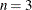parameters and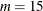functions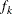: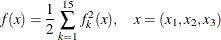where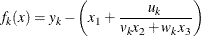with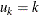,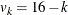,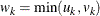, and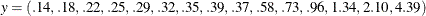The minimum function value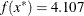E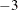is at the point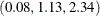. The starting point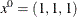is used.

The following is the naive way of specifying the objective function.

proc nlp tech=levmar;
lsq y1-y15;
parms x1-x3 = 1;
tmp1 = 15 * x2 + min(1,15) * x3;
y1 = 0.14 - (x1 + 1 / tmp1);
tmp1 = 14 * x2 + min(2,14) * x3;
y2 = 0.18 - (x1 + 2 / tmp1);
tmp1 = 13 * x2 + min(3,13) * x3;
y3 = 0.22 - (x1 + 3 / tmp1);
tmp1 = 12 * x2 + min(4,12) * x3;
y4 = 0.25 - (x1 + 4 / tmp1);
tmp1 = 11 * x2 + min(5,11) * x3;
y5 = 0.29 - (x1 + 5 / tmp1);
tmp1 = 10 * x2 + min(6,10) * x3;
y6 = 0.32 - (x1 + 6 / tmp1);
tmp1 = 9 * x2 + min(7,9) * x3;
y7 = 0.35 - (x1 + 7 / tmp1);
tmp1 = 8 * x2 + min(8,8) * x3;
y8 = 0.39 - (x1 + 8 / tmp1);
tmp1 = 7 * x2 + min(9,7) * x3;
y9 = 0.37 - (x1 + 9 / tmp1);
tmp1 = 6 * x2 + min(10,6) * x3;
y10 = 0.58 - (x1 + 10 / tmp1);
tmp1 = 5 * x2 + min(11,5) * x3;
y11 = 0.73 - (x1 + 11 / tmp1);
tmp1 = 4 * x2 + min(12,4) * x3;
y12 = 0.96 - (x1 + 12 / tmp1);
tmp1 = 3 * x2 + min(13,3) * x3;
y13 = 1.34 - (x1 + 13 / tmp1);
tmp1 = 2 * x2 + min(14,2) * x3;
y14 = 2.10 - (x1 + 14 / tmp1);
tmp1 = 1 * x2 + min(15,1) * x3;
y15 = 4.39 - (x1 + 15 / tmp1);
run;


A more economical way to program this problem uses the DATA= option to input the 15 terms in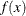.

data bard;
input r @@;
w1 = 16. - _n_;
w2 = min(_n_ , 16. - _n_);
datalines;
.14  .18  .22  .25  .29  .32  .35  .39
.37  .58  .73  .96 1.34 2.10 4.39
;

proc nlp data=bard tech=levmar;
lsq y;
parms x1-x3 = 1.;
y = r - (x1 + _obs_ / (w1 * x2 + w2 * x3));
run;


Another way you can specify the objective function uses the ARRAY statement and an explicit do loop, as in the following code.

proc nlp tech=levmar;
array r .14  .18  .22  .25  .29  .32  .35  .39  .37  .58
.73  .96 1.34 2.10 4.39 ;
array y y1-y15;
lsq y1-y15;
parms x1-x3 = 1.;
do i = 1 to 15;
w1 = 16. - i;
w2 = min(i , w1);
w3 = w1 * x2 + w2 * x3;
y[i] = r[i] - (x1 + i / w3);
end;
run;